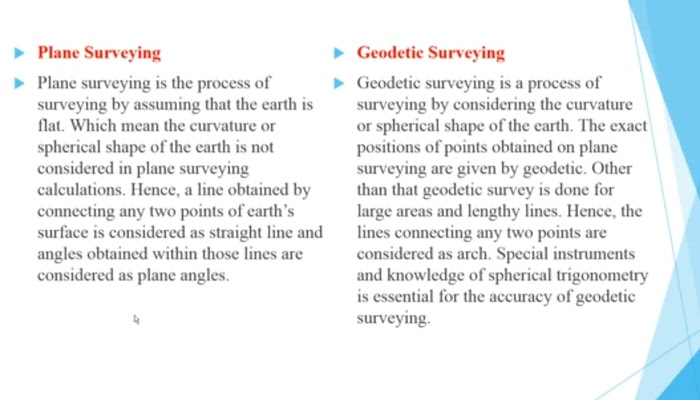# Difference Between Plane And geodetic surveying in civil engineering

In this article we are going to see about difference between plane surveying and geodytic surveying. Plane surveying is the process of surveying by assuming that the earth is flat which means the curvature or spherical shape of the earth is not considered in plane surveying calculations. Hence a line obtained by connecting any two points of earth surface is considered as straight line and angles obtained within those lines are considered as plane angles.

# In geodetic surveying

geodetic is a process of surveying by considering the curvature or spherical shape of the earth. The exact position of points obtained on planes surveying are given by geodetic. Other than the geodetic survey is done for large areas and lengthy lines. Hence the lines connecting any two points are considered as arch. Special instruments and knowledge of spherical trigonometry is essential for.

Across of geodetic surveying. Next we are going to see about difference between plane surveying and geodetic surveying. Plane surveying earth surface is assumed as a plane. Geodetic surveying earth surface is considered as spherical line formed by any two points are considered as straight line as the same angles or plane angles of the types bonds.

## Plane Surveying

Line formed by joining any two points are considered as arch as the same angles or spherical angles in plane surveying suitable for small area surveying. In geodytic surveying suitable for large area surveying, survey accuracy is low in plane surveying and survey accuracy is high for geodytic surveying. Economical and easy survey method.

Special in so much needed and long survey method. These are the points are undertaken for difference between plane and geotetic surveying. If you want to know more details about civil engineering visit ww.civilsnapsart.com for more details.Tags